• Account
• API
• Integrations
• Enterprise
• Resources
Number field type
• 01 Dec 2022
• Dark
Light

# Number field type

• Dark
Light

The number field type is a field type designed to hold numbers. The number field type is a general-purpose field type for most numerical values, like the number of chairs of a particular type your furniture business has in stock, or the distance from one city to another.

## Overview

Number fields should be used in cases where the values are referenced for computation or visualization, such as in formulas performing arithmetic or for plotting in the Chart extension. Number fields are not recommended for storing arbitrarily long strings that happen to be numeric, such as account numbers, because larger numbers (above 15 digits) will be rounded. Instead, consider using a text field in these cases.

Additionally, there are other numeric field types that might be a better fit for your values depending on the types of numeric values you're trying to store. For example:

• If you're looking to store a time duration measured in hours, minutes, seconds, or milliseconds, try the duration field type, which will format your values in a duration format like h:mm:ss.
• If you're looking to store an amount of money, try the currency field type, which will prefix the numbers in your field with the currency symbol of your choice, e.g. ₩1000.
• If you're looking to store a number as a percent, try the percent field type, which will express your number as a fraction of 100 denoted with the percent sign, e.g. 45%.

## Format

When customizing your number field, the Format tab of the field customization menu contains a couple of options.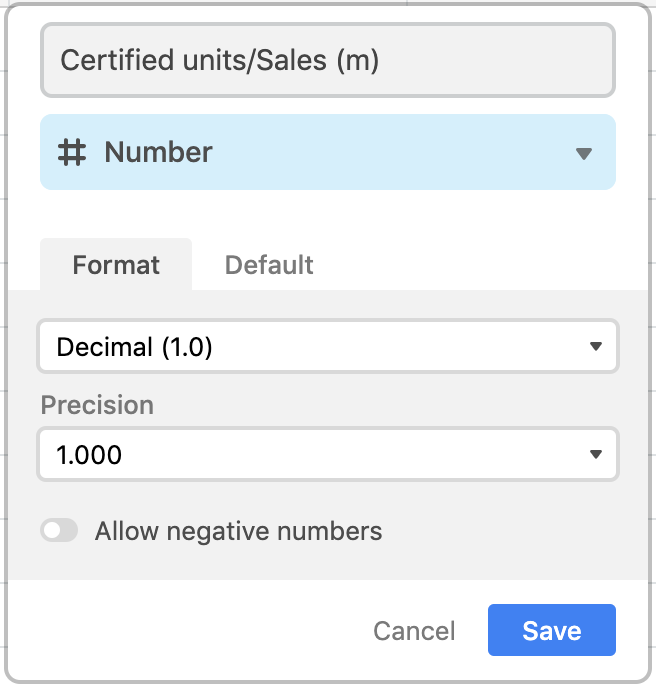You can select whether you want your numbers to appear as integers (whole numbers) or as decimal numbers.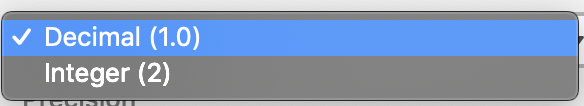If you've chosen to format your numbers as decimals, you'll also have the option of selecting the precision, up to 8 decimal places.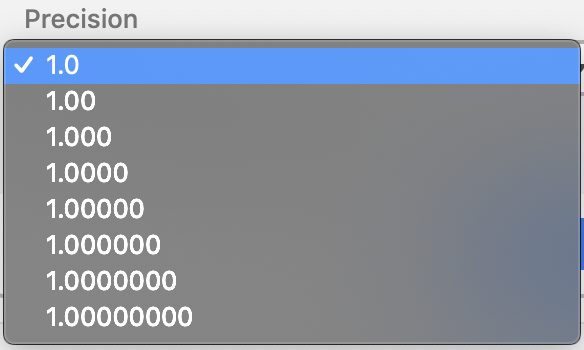You can also decide whether or not you want to allow negative numbers in this field.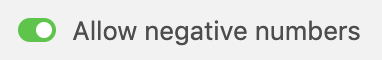## Show percent sign (legacy)

Note : this formatting option only appears in certain fields created before May 2019. For fields created after May 2019, you should use the percent field type if you want to format numbers as percentages.

Legacy percent fields created before May 2019 were converted into number field types containing the Show percent sign toggle.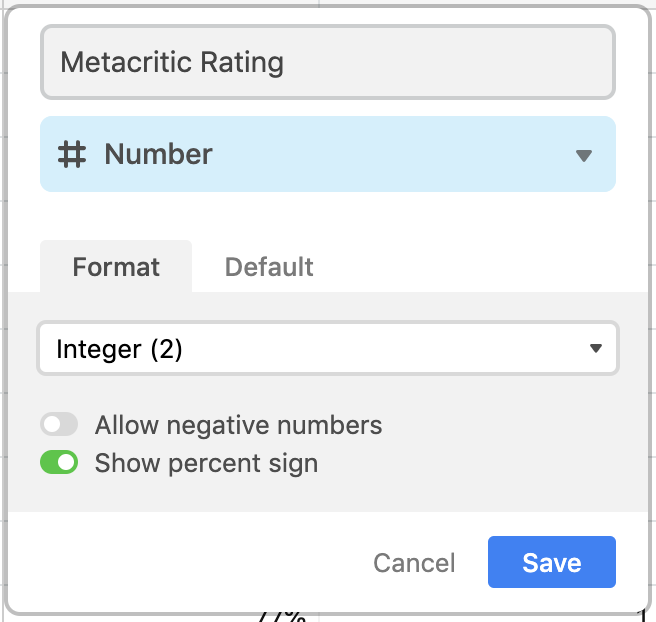This toggle, when checked, formats the cells in this field to display a percent sign (%) at the end of the number value in each cell.

For example, the value 76 in a cell will appear as 76%. However, this percent sign is essentially cosmetic, as the underlying value contained in the cell is 76, not 76/100.

### Converting from a number field to a percent field

IMPORTANT: If you choose to convert a number field into a percent field while the Show percent sign is toggled on, this will divide any values in your field by 100.

For example, if a cell in a number field contains the number 76 (displayed as 76% with the Show percent sign toggle on), and then you change the field type to percent, then after conversion, the cell value will still appear as 76%. However, the underlying value in the cell will be changed from 76 to 76/100, i.e., .76.

Please be aware that making this conversion may have effects on some aspects of your base:

• Formulas (if the formula incorporates the value of the field into its output)
• Record coloring (if your coloring conditions are determined by values in a field)
• Filters (if your filter conditions are determined by values in the field)
• View share links (if the records visible in the share link are determined by filters)
• API integrations (if your API integration relies on the values returned by the field)

Note that the division will NOT happen if you convert a standard number field that does not contain a Show percent sign toggle—instead, the values will remain the same, but be formatted differently. So, for example, if you convert a standard number field containing the value 76 into a percent field, the cell value will appear as 7600%.

## FAQs

#### Is there a way to display numbers using a comma, instead of a decimal?

There is no universal setting to change this, but you can use a formula field to mimic the behavior. Here's an example: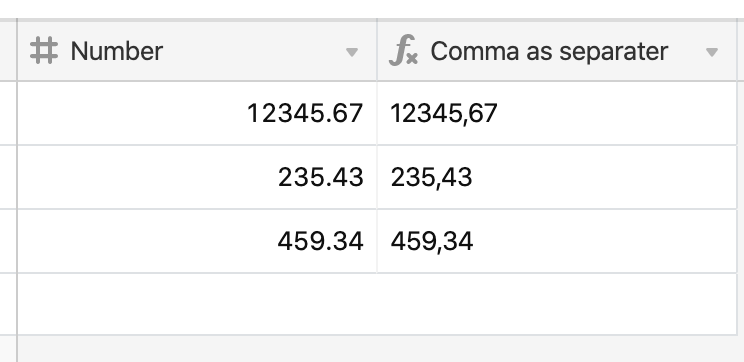In the above example we created a formula field and used the below formula to substitute all decimals contained in the "Number" field with commas:

``````
SUBSTITUTE(CONCATENATE({Number}), '.', ',')
``````

If you use the above formula in your workflow—make sure to update the field name from "Number" to the field you'd like to replace the decimals in.

#### An API call is returning different numbers than are shown in the Airtable API, why might this be?

A Number field can be formatted as an Integer in the UI but this does not guarantee the number is returned by the API as a whole number integer. It can be returned as a float (i.e. 3.2424 instead of 3). The parameter `cellFormat=string` should be used to cast the integer to a string then the client should cast back to an integer. To learn more about this parameter, visit the Web API documentation.